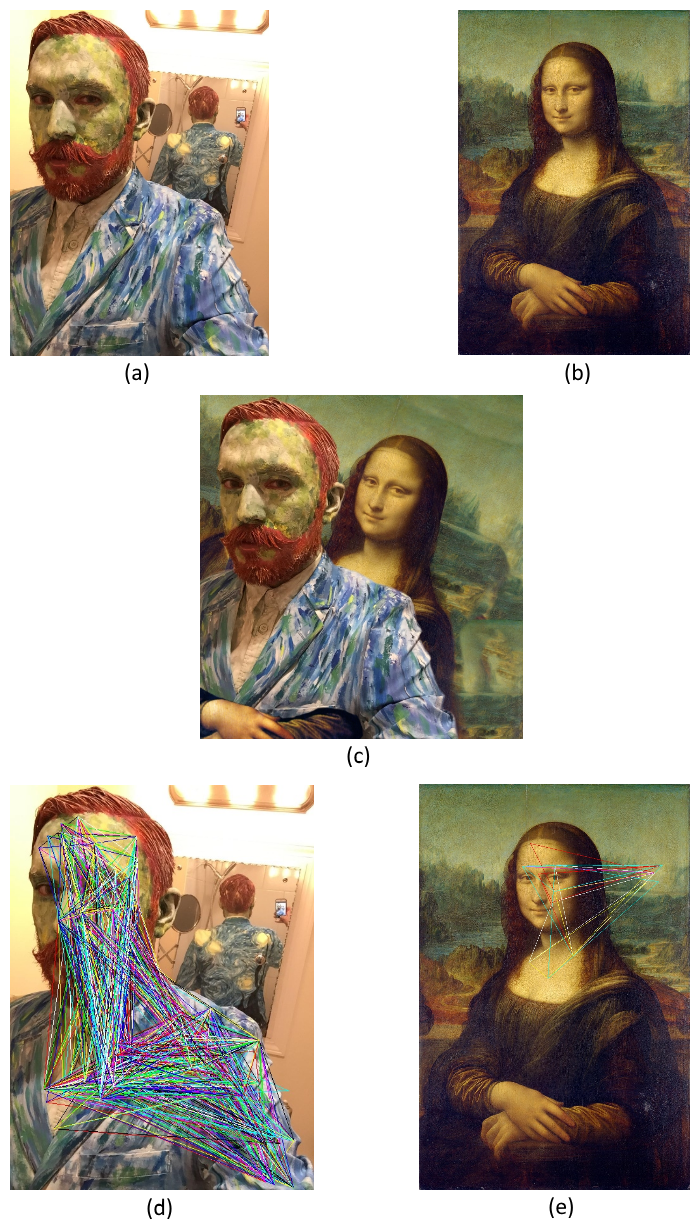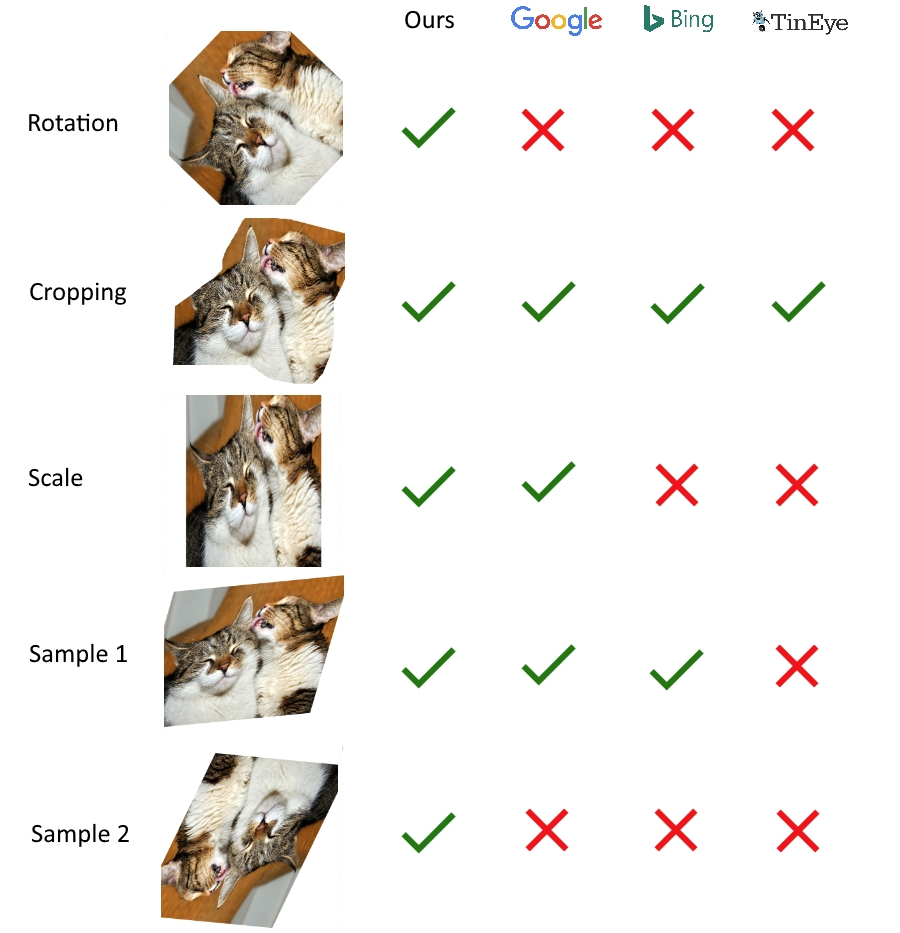Image Matching Demo

Click and drag on the query image to apply a transformation.

This demo showcases a reverse image search algorithm which performs 2D affine transformation-invariant partial image-matching in sublinear time. The algorithm compares an input image to its database of preprocessed images and determines if the input matches any image in the database. The database need not contain the original image as inputs can be matched to any 2D affine transformation of the original. This means that images which have been scaled (uniformly or non-uniformly), skewed, translated, cropped or rotated (or have undergone any combination of these transformations) can be identified as coming from the same source image (Figure 1).

The algorithm runs in sublinear time with respect to the number of images in the database regardless of the number of transformations applied. Note that if image-matching could not be done in sublinear time it would not function at the scale that the likes of Google or Microsoft require.

Figure 1. 2D affine transformation invariant image-matching

If the input is a composite of images or image fragments, the algorithm will return matches for each image/image fragment (Figure 2).Figure 2. The query image (c), which is a composite of (a) and (b), matches the two images (d) and (e) stored in the database. The code to reproduce this result can be found here.

How it Works

1.
The algorithm finds keypoints in the input using edge detection1.
2.
Each set of three keypoints is converted into a triangle2.
3.
These triangles are transformed into equilateral triangles.
4.
Each equilateral triangle is rotated to each of it's 3 edges and a perceptual hashing algorithm (in this case PHash) is used to produce a hash for each side3.
5.
The algorithm compares the hash to those stored in the database and returns all matching images4.

All images in the database have been preprocessed in this manner to produce hashes for comparison.

Figure 3. Step-by-step video guide showing how the algorithm operates
1
Any keypoint-finding algorithm can be used so long as it is 2D affine transformation-invariant.
2
The comparison can be done through a hash lookup (which can be done in constant time with respect to the number of images in the database) or by finding a ‘nearest-neighbour’ in the database (which can be done in amortized O(log2n) time).
3
Rotating the triangle to each of it's 3 sides and hashing each rotation keeps the algorithm rotation invariant.
4
The algorithm will return multiple matches if the input is a composite of images.

How it Compares to the Competition

As you can see in Figure 4 below, the algorithm performs better than industry leaders in matching images which have undergone 2D affine transformations. Even the best-performing service, Google Image Search, fails to handle a simple 45 degree rotation.Figure 4. Comparison of the image-matching capabilities of our algorithm versus market leaders. The code to reproduce this result can be found here.

Market leaders show limited ability to find matches of images which have undergone certain transformations. Our algorithm solves this problem for 2D affine transformations and, if used in conjunction with other modern techniques, offers a significant improvement in reverse-image searching.